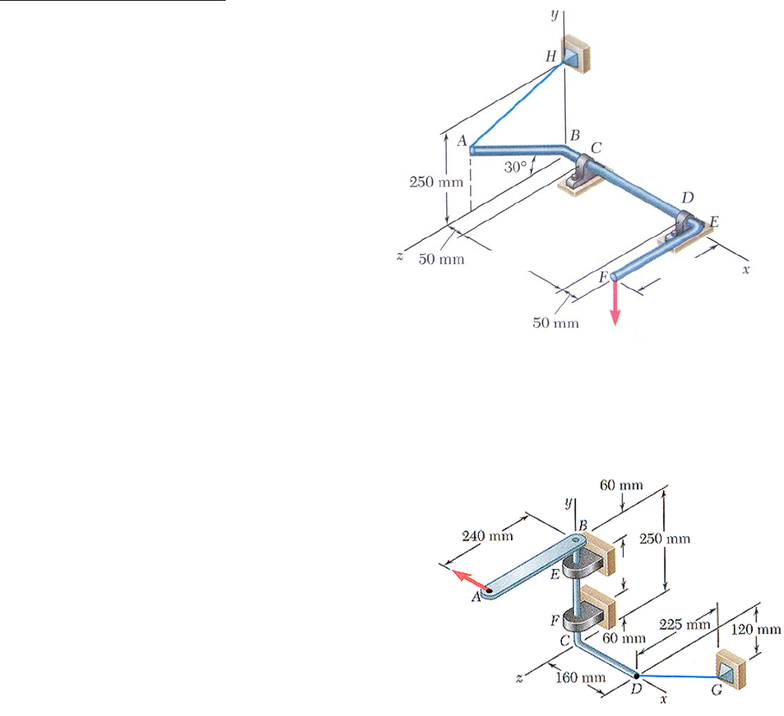# ENGG 202 Lecture Notes - Lecture 1: Wind Engineering, Ferrari 250

120 views4 pagesENGG 202 Tutorial 10 March 19/20, 2013
P
300 mm 250 mm
3D Equilibrium of Rigid Bodies
1) The bent rod ABEF is supported in the position
shown by bearings at C and D and by wire AH.
Neither bearing is capable of exerting couples. The
bearing at D is not capable of exerting axial thrust,
while the bearing at C is. The force P = 400 N and
is parallel to the y- axis. Assume the weight of the
rod is negligible. Portion AB of the rod is 250 mm
long. a) Write the one equation of equilibrium that
could be used to find the tension in the cable AH
directly? ANS: ΣMCD = 0 b) Determine the support
reactions at bearings C and D and the tension in the
format.
ANS:T=230.9 j + -400.0 k N, D = 505.2 j + -66.7 k N, C = -336.1 j + 466.7 k
N
Solution Strategy: Draw FBD of the bar ABCDEF. There are 2 force reactions at D (y and z), 3
force reactions at C, and one unknown cable tension for a total of 6 unknowns. A) By summing
moments about line CD, the force in the cable could be found directly (1 eqn 1 unknown). B)
Using vectors, sum the moments about C = 0 to obtain 3 eqns of equil and solve for reactions at
D and the cable force. Then write the force equil eqns to solve for reactions at C.
2) The lever AB is welded to the bent rod BCD which is
supported by bearings at E and F and by cable DG. The
cable DG lies in a y-z plane. The force P is 330 N and is
parallel to the x-axis. Knowing that both bearings are
properly aligned (and therefore cannot exert
couples/moments) and only the bearing at E does not exert
any axial thrust, determine (a) the tension in cable DG (b)
the reactions at bearings E and F
ANS: a) 561 N b) Ez=-228.4 N, Ex=157 N, Fx=173.1 N, Fy=264.3N, Fz=723.6N
Solution Strategy: Draw FBD of the assembly
ABEFCD. There are 2 force reactions at E (x and z), 3
force reactions at F, and one unknown cable tension for
a total of 6 unknowns. Using vectors, sum the moments about F = 0 to obtain 3 eqns of equil and
solve for reactions at E and the cable force. Then write the force equil eqns to solve for reactions
at F.
P
Unlock document

This preview shows page 1 of the document.
Unlock all 4 pages and 3 million more documents.